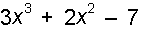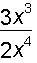﻿ Entering Equations

# Entering Equations

Top  Previous  Next
 Equations are entered into the equation tool by typing what you see WITHOUT formatting.  On most occasions the equation tool will automatically format your equation for you. For example.  If you want the equationsimply load the equation tool and enter 3x3+2x2-7.  The equation tool automatically formats the powers and italicizes the variables.   Simple fractions are also entered as you see them.  In other words if you wantyou enter it as 3x3/2x4 and The equation tool will automatically build the appropriate fraction.  Most simple equations can be entered this way, with NO formatting on your part.  More complicated equations will require some extra input on your part.